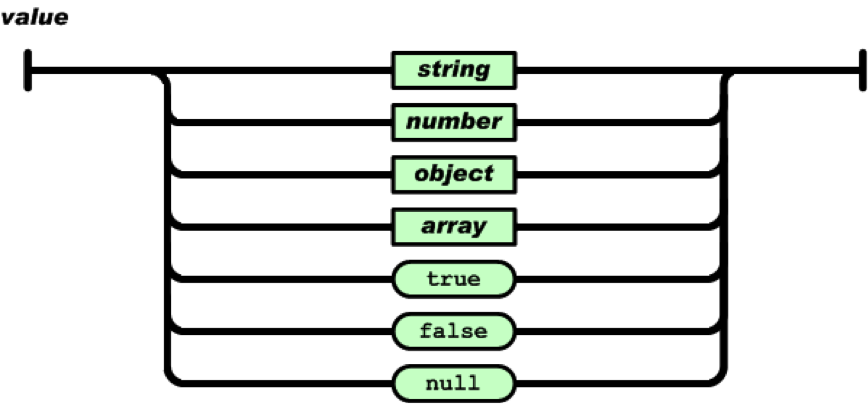Json是平时常用的数据结构，对于C++ Native代码来说，选择一个优秀的json解析器对于应用来说十分重要。json_spirit是codeproject上比较热门的json解析器，具有优异的使用接口，以及不错的效率（用了boost::spirit::classic解析框架）。

## 先简单介绍JSON语法（JavaScript Object Notation）

1. “名称/值”对的集合（A collection of name/value pairs），它被理解为对象（object）或字典（dictionary）
2. 值的有序列表（An ordered list of values），它被理解为数组（array）

### 对象

• 一个对象以“{”（左括号）开始，“}”（右括号）结束
• 每个“名称”后跟一个“:”（冒号）
• “‘名称/值’ 对”之间使用“,”（逗号）分隔。### 数组### 值

（value）可以是双引号括起来的字符串（string）、数值(number)、true、false、 null、对象（object）或者数组（array）。因此可以递归嵌套。## json spirit部分源码分析

json_spirit源码参考

### value的结构

``````enum Value_type{ obj_type, array_type, str_type, bool_type, int_type, real_type, null_type };

// 这个Value_type只是标识哪种类型
// Config 配置决定使用wstring或string以及map或者vector来实现
template< class Config >
class Value_impl
{
public:
typedef Config Config_type;
typedef typename Config::String_type String_type;// 这些typedef只是转发了Config的类型
typedef typename Config::Object_type Object;
typedef typename Config::Array_type Array;
typedef typename String_type::const_pointer Const_str_ptr;  // eg const char*

Value_impl();  // creates null value

// 构造函数支持接受的类型，因为内部使用了boost::variant
Value_impl( Const_str_ptr      value );
Value_impl( const String_type& value );
Value_impl( const Object&      value );
Value_impl( const Array&       value );
Value_impl( bool               value );
Value_impl( int                value );
Value_impl( boost::int64_t     value );
Value_impl( boost::uint64_t    value );
Value_impl( double             value );
Value_impl( const Value_impl& other ); // 支持复制构造

bool operator==( const Value_impl& lhs ) const;
Value_impl& operator=( const Value_impl& lhs ); // 支持赋值
Value_type type() const;
bool is_uint64() const;
bool is_null() const;

// 获取当前值，当然你是知道要获取的类型的，类型不匹配时抛出异常（基于variant的实现）
const String_type&  get_str()    const;
const Object&      get_obj()    const;
const Array&       get_array()  const;
bool               get_bool()   const;
int                get_int()    const;
boost::int64_t       get_int64()  const;
boost::uint64_t      get_uint64() const;
double             get_real()   const;
Object& get_obj(); // 注意返回非常量引用可以直接修改原内容
Array&  get_array();

// get_value提供一个统一的接口，取得你想要的类型的值 Type val = val_impl. get_value< Type >();
template< typename T > T get_value() const;

static const Value_impl null;

private:
// 这个Variant是基于递归包装辅助类recursive_wrapper来实现的
// 从语法可以看到Variant可以是普通的值，也可以是数组或者对象，而数组或对象是可以基于value来组成的，从而导致循环依赖。
typedef boost::variant< String_type,
boost::recursive_wrapper< Object >, boost::recursive_wrapper< Array >,
bool, boost::int64_t, double > Variant;

// 三个数据成员
Value_type type_;	// 表示类型
Variant v_;	// 这个variaant对象存储着当前对象的类型信息和值。
bool is_uint64_;
};
``````

### 配置Config的说明

``````template< class String > // String配置支持wstring 或者 string
struct Config_map
{
typedef String String_type;

// 配置真正的value类型，基于Value_impl实现
typedef Value_impl< Config_map > Value_type;

//数组是vector< Value_type >
typedef std::vector< Value_type > Array_type;

// 对象的类型 map
typedef std::map< String_type, Value_type > Object_type;
typedef typename Object_type::value_type Pair_type;

// 以下的三个静态函数只是用于语义动作时绑定使用的。对我们没有使用价值
// 如果基于Qi实现，直接使用phoenix来做语义动作，而无需到处分散的函数。
const String_type& name, const Value_type& value )
{
return obj[ name ] = value;
}
static String_type get_name( const Pair_type& pair )
{
return pair.first;
}
static Value_type get_value( const Pair_type& pair )
{
return pair.second;
}
};
``````

### 语法的大致情况

(1)、Semantic_actions类来专门封装一个语义动作类。以下是它的成员:

``````Value_type& value_;             // 对象或数组
Value_type* current_p_;         // 当前被创建的对象或数组
vector< Value_type* > stack_;   // 维持的一个船舰对象或数组的栈
String_type name_;              // of current name/value pair
``````

(2)、核心的语法定义

``````template< typename ScannerT >
class definition
{
public:
definition( const Json_grammer& self )
{
//...

// actual grammer
json_
= value_ | eps_p[ &throw_not_value ]
;
value_		// value 的定义，可以嵌套为object
= string_[ new_str ]
| number_
| object_
| array_
| str_p( "true" ) [ new_true  ]
| str_p( "false" )[ new_false ]
| str_p( "null" ) [ new_null  ]
;
object_
= ch_p('{')[ begin_obj ]
>> !members_
>> ( ch_p('}')[ end_obj ] | eps_p[ &throw_not_object ] )
;
members_
= pair_ >> *( ',' >> pair_ )
;
pair_			// 要放入map的pair: name/value 对
= string_[ new_name ]
>> ( ':' | eps_p[ &throw_not_colon ] )
>> ( value_ | eps_p[ &throw_not_value ] )
;
array_
= ch_p('[')[ begin_array ]
>> !elements_
>> ( ch_p(']')[ end_array ] | eps_p[ &throw_not_array ] )
;
elements_
= value_ >> *( ',' >> value_ )
;
string_
= lexeme_d // this causes white space inside a string to be retained
[
confix_p
(
'"',
*lex_escape_ch_p,
'"'
)
]
;
number_
= strict_real_p[ new_real   ]
| int64_p      [ new_int    ]
| uint64_p     [ new_uint64 ]
;
}
rule< ScannerT > json_, object_, members_, pair_, array_, elements_, value_, string_, number_;
const rule< ScannerT >& start() const { return json_; } //语法起点
};
``````

## 理解json spirit的使用及注意事项

### 解析json字符串

``````typedef Config_map< std::wstring > wmConfig;
typedef wmConfig::Value_type  wmValue;
typedef wmConfig::Object_type wmObject;
typedef wmConfig::Array_type  wmArray;
``````

``````// 配置真正的value类型，基于Value_impl实现
typedef Value_impl< Config_map > Value_type;

//数组是vector< Value_type >
typedef std::vector< Value_type > Array_type;

// 对象的类型 map
typedef std::map< String_type, Value_type > Object_type;
``````
• wmValue`Value_impl< Config_map< std::wstring > >`，从wmValue对象可以get得到真正包含在variant中的对象。它本身是variant对象。
• wmObject`map< wstring, wmValue >` 与传统的map不一样，由于值是variant型，可以为指定的任意类型
• wmArray`vector< wmValue >` ，也与普通vector的“单类型”不一样。

``````bool read( const std::wstring& s, wmValue& value );// 从字符串取得json数据
bool read( std::wistream&  is, wmValue& value );//从（文件）流去的数据
``````

### 生成json字符串

``````void write( const wmValue& value, std::wostream& os );
void write_formatted( const wmValue& value, std::wostream& os );
std::wstring write( const wmValue& value );
std::wstring write_formatted( const wmValue& value );
``````